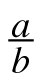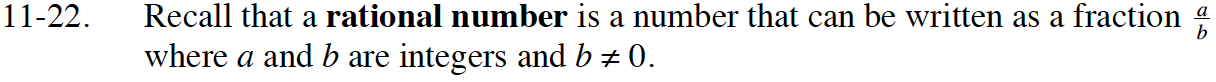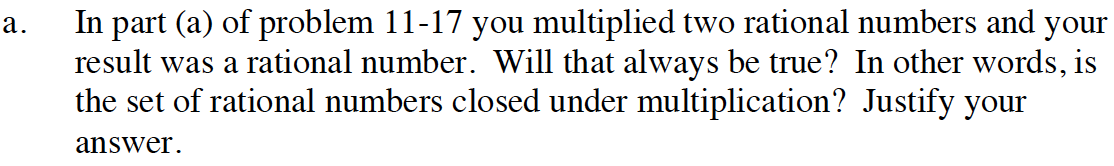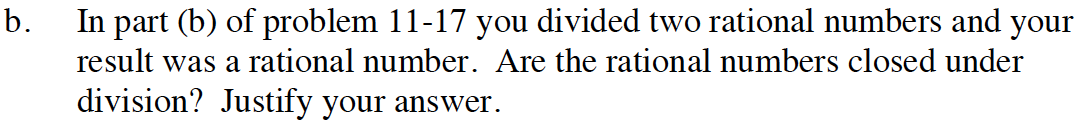Home > INT3 > Chapter 11 > Lesson 11.1.2 > Problem11-22

11-22.
1. Recall that a rational number is a number that can be written as a fractionwhere a and b are integers and b ≠ 0. Homework Help ✎

1. In part (a) of problem 11‑17 you multiplied two rational numbers and your result was a rational number. Will that always be true? In other words, is the set of rational numbers closed under multiplication? Justify your answer.

2. In part (b) of problem 11-17 you divided two rational numbers and your result was a rational number. Are the rational numbers closed under division? Justify your answer.Is this answer a rational number?Use the same method as part (a).